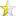• Articles
• Dynamically sorting both keys and values
Sep 24, 2014 (last update: Oct 30, 2014)

# Dynamically sorting both keys and valuesWell, you might have faced this problem in your coding life. Also you might know the answer already. The problem was about the std::map or say std::multimap, both of them are special type of containers who can sort the items by a comparing function pointer(or a built-in one like std::less). They sort it when you are inserting a new item, that's of course so handy, because you don't wanna call a sorting function every time you need to! Actually you will never explicitly call any sorting function on your map or multimap, coz it's already sorted! Now here's my problem-
Say, I have a map like this -
```D - 1
D - 2
B - 3
A - 4
```

Now I need to sort them like this -
```A - 4
B - 3
D - 2
D - 1
```
Here's the explanation of this sorting- first elements(A,B,D) will be sorted in ascending order A --> B --> D regardless of the second element, secondly if the first elements are equal (i.e 2 Ds here) then they will be sorted as the descending order of their corresponding second element.
Using std::multimap will end-up with only the first sorting part, so I took advantage of templates and inheritances. Here's the code -
 ``12345678910111213141516171819202122232425262728293031323334353637`` ``````#include #include #include using namespace std; template > class MMap : public set < pair< _A, _B >, _Compare > { public : MMap():set< pair< _A, _B >, _Compare >(){}; ~MMap(){}; }; template< typename InPair > struct MMapComp{ bool operator() (InPair a , InPair b){ if( a.first == b.first ) return a.second > b.second; else return a.first < b.first; } }; int main(int argc, char ** argv) { MMap > > test; test.insert(make_pair('D',1)); test.insert(make_pair('D',2)); test.insert(make_pair('B',3)); test.insert(make_pair('A',4)); for( MMap::iterator it = test.begin(); it != test.end(); it++ ) cout << (*it).first << "\t" << (*it).second << endl; return 0; } ``````

And here's the output -
 ``` A 4 B 3 D 2 D 1 ```

Ok, I apologize for my undocumented code :) But it's not so hard to read actually. Is it ?